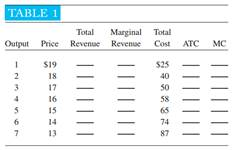### Create an Account

Home / Questions / Fill in Table 1 Using your own piece of graph paper draw a graph of the firm’s demand, m...

# Fill in Table 1 Using your own piece of graph paper draw a graph of the firm’s demand, marginal revenue marginal cost and average total cost curves Calculate the firm’s total profit

( a ) Fill in Table 1. (b) Using your own piece of graph paper, draw a graph of the firm’s demand, marginal revenue, marginal cost, and average total cost curves. (c) Calculate the firm’s total profit. (d) If the firm operates at optimum efficiency, how much will its output be? (e) If the firm were a perfect competitor, how much would its price be in the long run?Apr 24 2020 View more View LessSubscribe To Get Solution